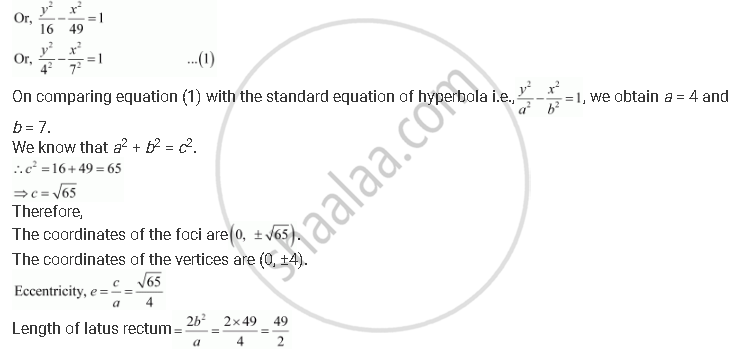CBSE (Arts) Class 11CBSE
Share
Notifications

View all notifications
Books Shortlist
Your shortlist is empty

# Find the Coordinates of the Foci and the Vertices, the Eccentricity, and the Length of the Latus Rectum of the Hyperbola 49y2 – 16x2 = 784 - CBSE (Arts) Class 11 - Mathematics

Login
Create free account

Forgot password?
ConceptHyperbola Eccentricity

#### Question

Find the coordinates of the foci and the vertices, the eccentricity, and the length of the latus rectum of the hyperbola 49y2 – 16x2 = 784

#### Solution

The given equation is 49y2 – 16x2 = 784.

It can be written as
49y2 – 16x2 = 784Is there an error in this question or solution?

#### APPEARS IN

Solution Find the Coordinates of the Foci and the Vertices, the Eccentricity, and the Length of the Latus Rectum of the Hyperbola 49y2 – 16x2 = 784 Concept: Hyperbola - Eccentricity.
S